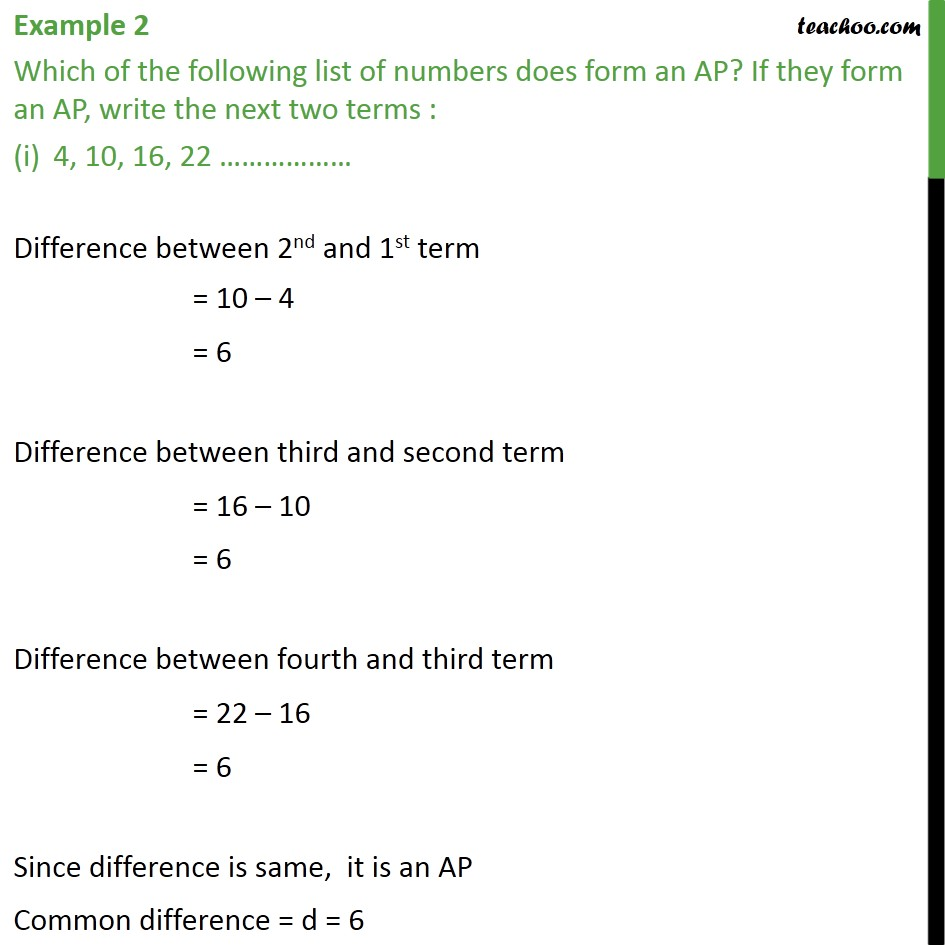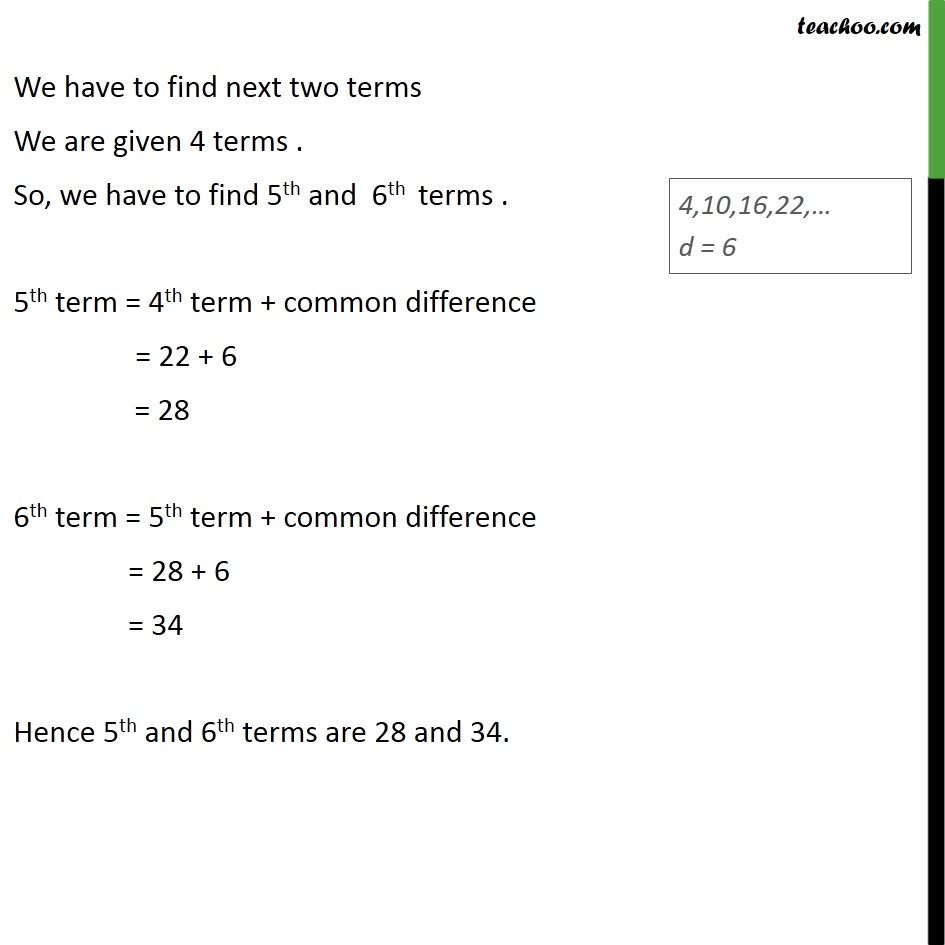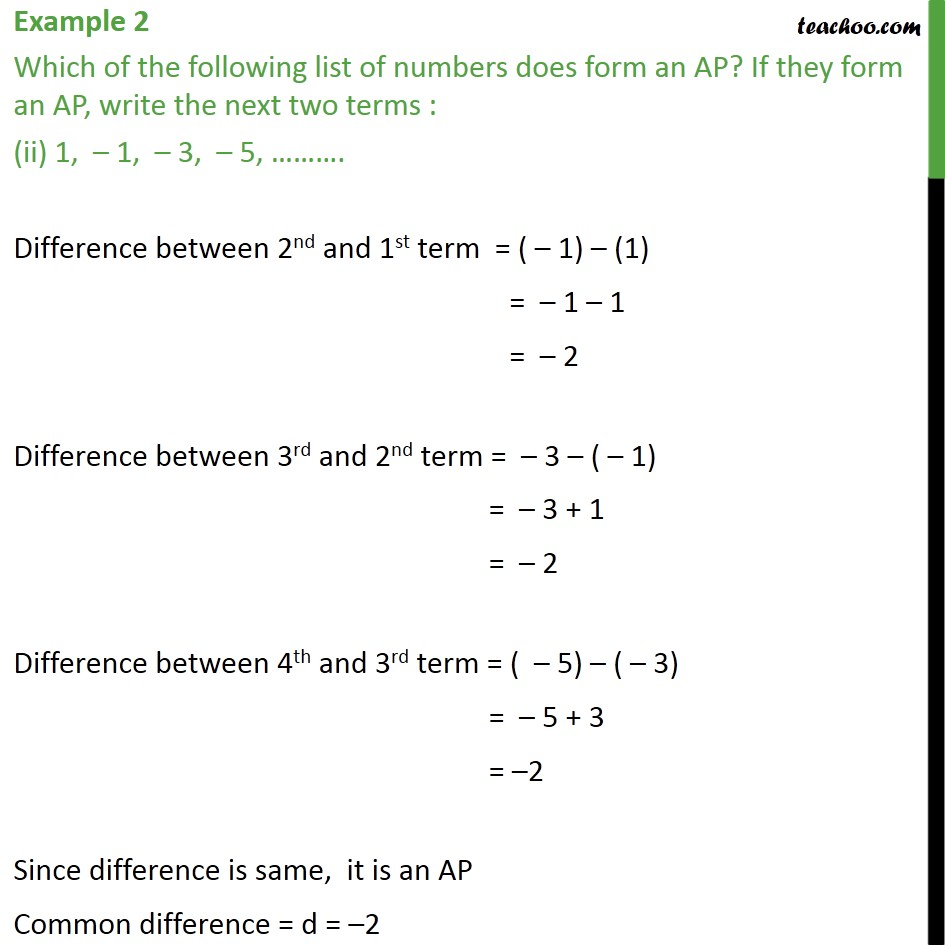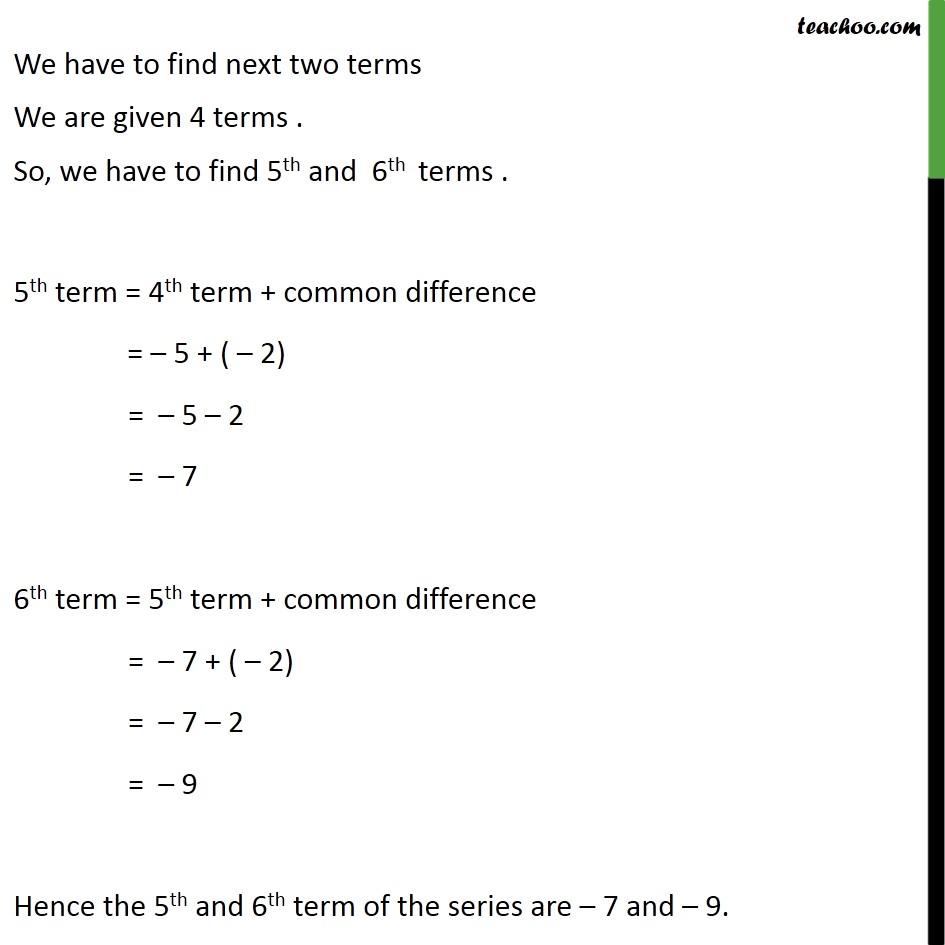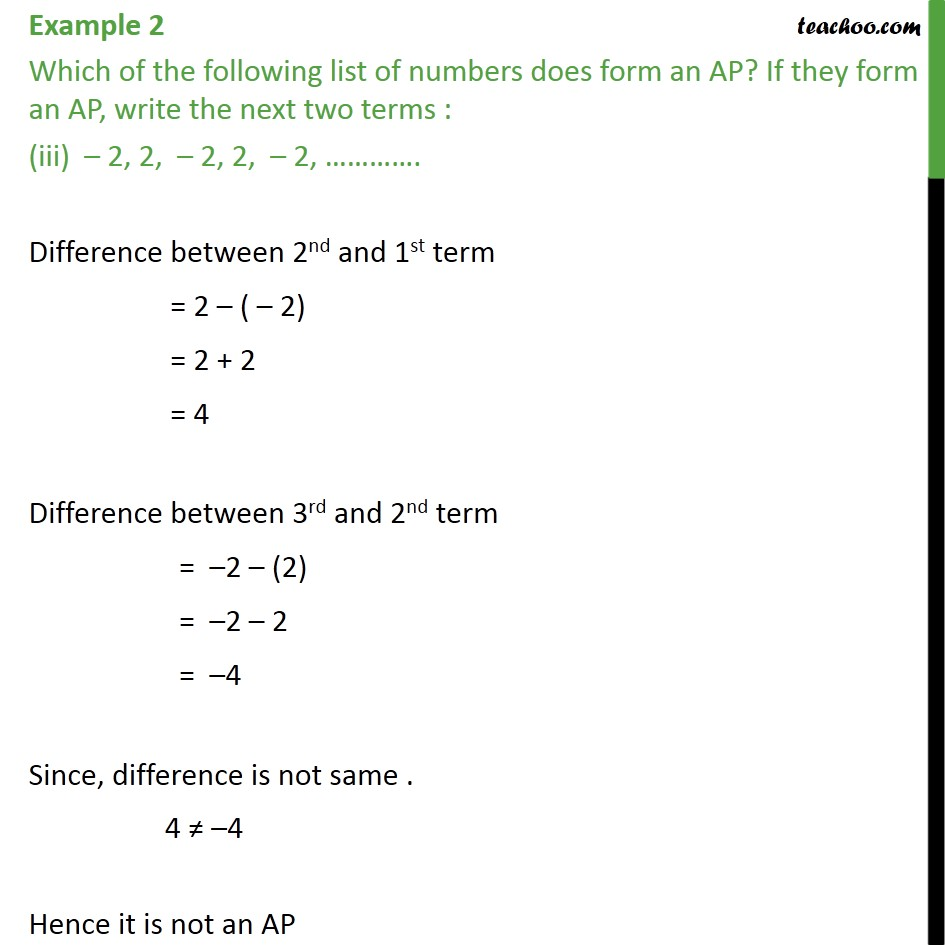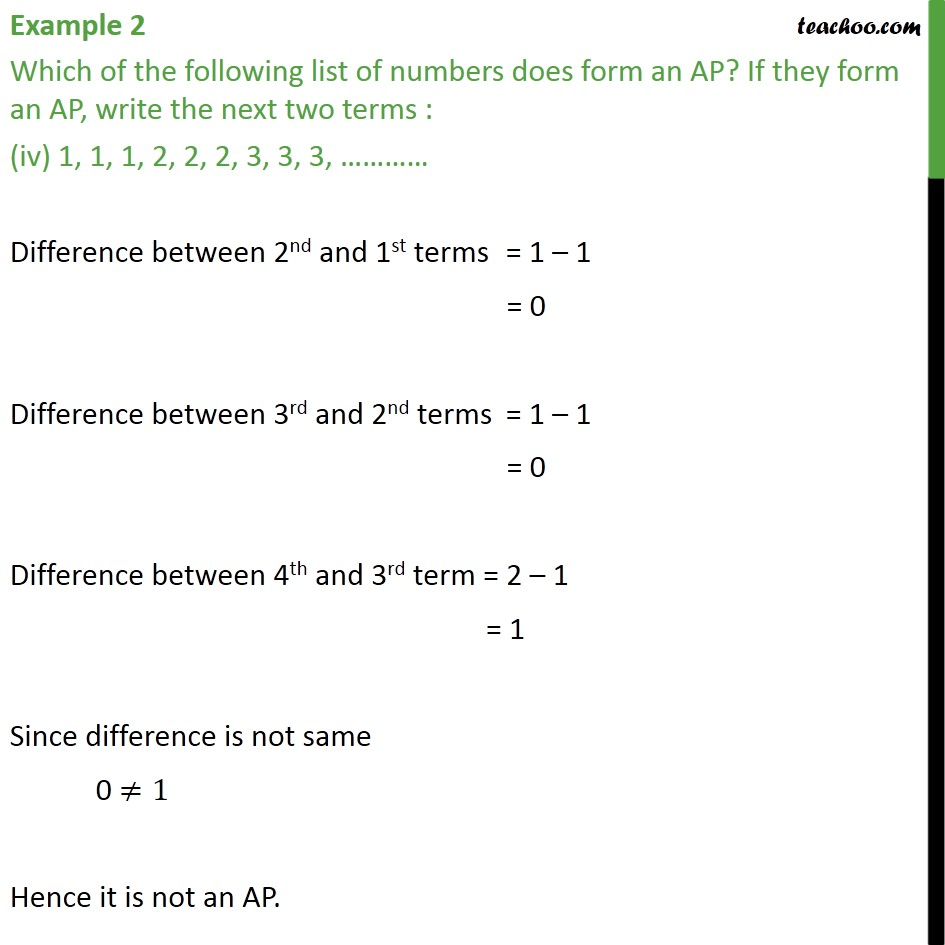1. Chapter 5 Class 10 Arithmetic Progressions
2. Concept wise
3. Checking if AP or not and finding a, d

Transcript

Example 2 Which of the following list of numbers does form an AP? If they form an AP, write the next two terms : 4, 10, 16, 22 Difference between 2nd and 1st term = 10 4 = 6 Difference between third and second term = 16 10 = 6 Difference between fourth and third term = 22 16 = 6 Since difference is same, it is an AP Common difference = d = 6 We have to find next two terms We are given 4 terms . So, we have to find 5th and 6th terms . 5th term = 4th term + common difference = 22 + 6 = 28 6th term = 5th term + common difference = 28 + 6 = 34 Hence 5th and 6th terms are 28 and 34. Example 2 Which of the following list of numbers does form an AP? If they form an AP, write the next two terms : (ii) 1, 1, 3, 5, . Difference between 2nd and 1st term = ( 1) (1) = 1 1 = 2 Difference between 3rd and 2nd term = 3 ( 1) = 3 + 1 = 2 Difference between 4th and 3rd term = ( 5) ( 3) = 5 + 3 = 2 Since difference is same, it is an AP Common difference = d = 2 We have to find next two terms We are given 4 terms . So, we have to find 5th and 6th terms . 5th term = 4th term + common difference = 5 + ( 2) = 5 2 = 7 6th term = 5th term + common difference = 7 + ( 2) = 7 2 = 9 Hence the 5th and 6th term of the series are 7 and 9. Example 2 Which of the following list of numbers does form an AP? If they form an AP, write the next two terms : (iii) 2, 2, 2, 2, 2, . Difference between 2nd and 1st term = 2 ( 2) = 2 + 2 = 4 Difference between 3rd and 2nd term = 2 (2) = 2 2 = 4 Since, difference is not same . 4 4 Hence it is not an AP Example 2 Which of the following list of numbers does form an AP? If they form an AP, write the next two terms : (iv) 1, 1, 1, 2, 2, 2, 3, 3, 3, Difference between 2nd and 1st terms = 1 1 = 0 Difference between 3rd and 2nd terms = 1 1 = 0 Difference between 4th and 3rd term = 2 1 = 1 Since difference is not same 0 1 Hence it is not an AP.

Checking if AP or not and finding a, d

About the AuthorDavneet Singh
Davneet Singh is a graduate from Indian Institute of Technology, Kanpur. He has been teaching from the past 9 years. He provides courses for Maths and Science at Teachoo.